# Elliptical Dipole Holger Witte Brookhaven National Laboratory Advanced

• Slides: 29
Download presentation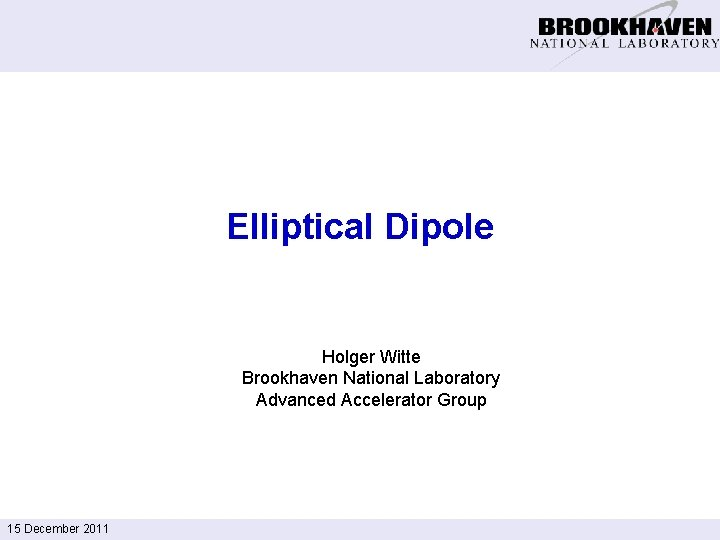Elliptical Dipole Holger Witte Brookhaven National Laboratory Advanced Accelerator Group 15 December 2011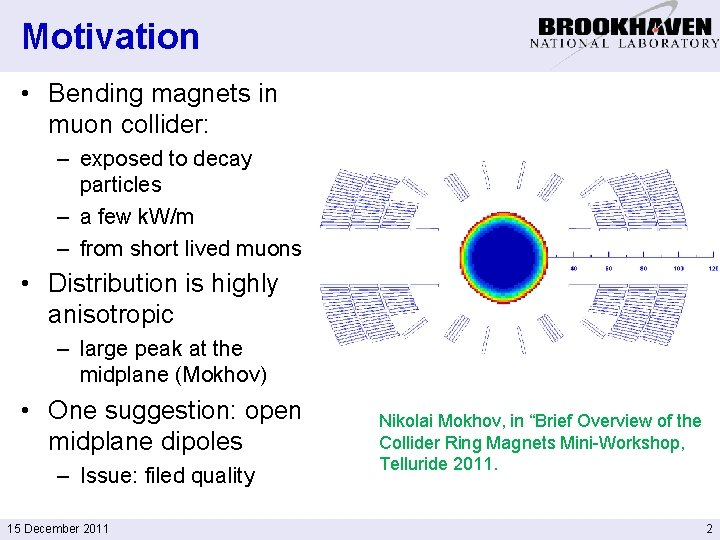Motivation • Bending magnets in muon collider: – exposed to decay particles – a few k. W/m – from short lived muons • Distribution is highly anisotropic – large peak at the midplane (Mokhov) • One suggestion: open midplane dipoles – Issue: filed quality 15 December 2011 Nikolai Mokhov, in “Brief Overview of the Collider Ring Magnets Mini-Workshop, Telluride 2011. 2Task Inside pipe width = 5 cm Inside pipe height = 2 cm Tungsten liner From: Suggested shield & cos theta dipole dimensions R. B. Palmer, 5/26/11 15 December 2011 3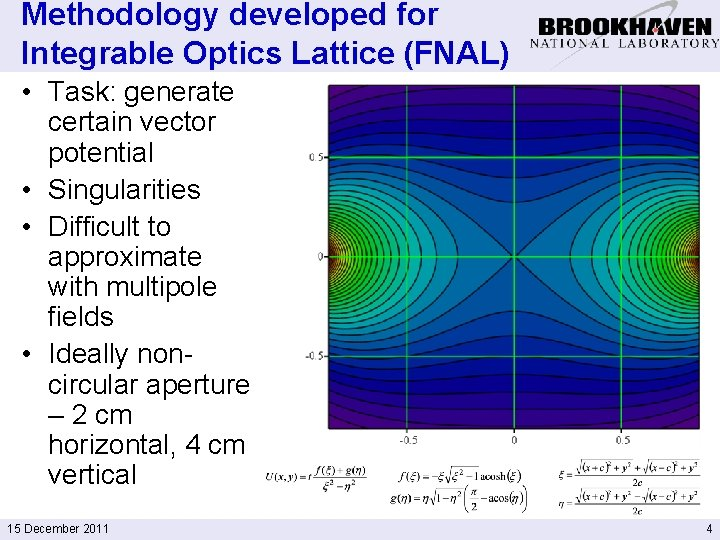Methodology developed for Integrable Optics Lattice (FNAL) • Task: generate certain vector potential • Singularities • Difficult to approximate with multipole fields • Ideally noncircular aperture – 2 cm horizontal, 4 cm vertical 15 December 2011 4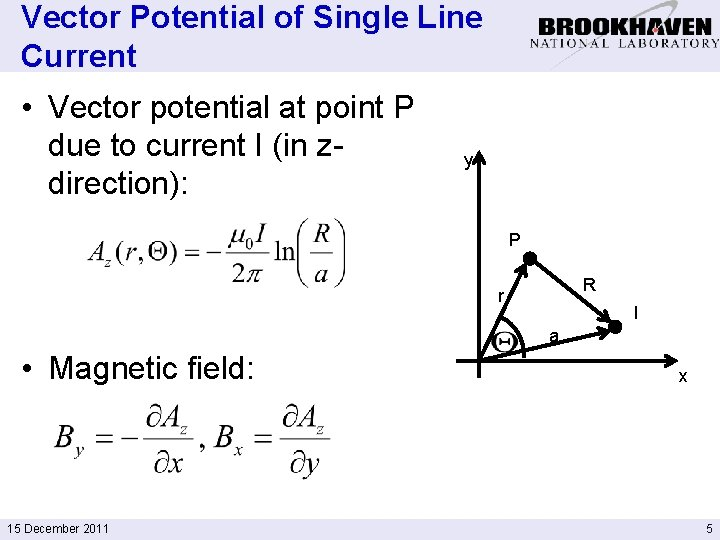Vector Potential of Single Line Current • Vector potential at point P due to current I (in zdirection): y P R r I a • Magnetic field: 15 December 2011 x 5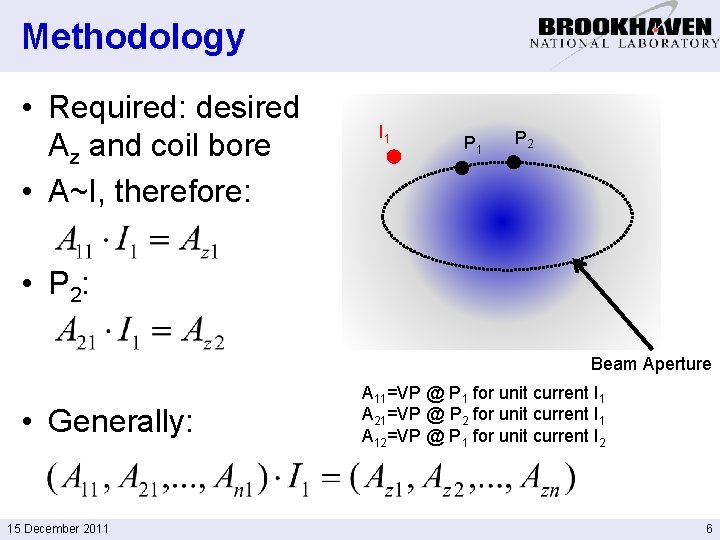Methodology • Required: desired Az and coil bore • A~I, therefore: I 1 P 2 • P 2: Beam Aperture • Generally: 15 December 2011 A 11=VP @ P 1 for unit current I 1 A 21=VP @ P 2 for unit current I 1 A 12=VP @ P 1 for unit current I 2 6Methodology: Formalism • Same is true for multiple currents and positions P • Formalism: A · x = I 1 b • Linear equation system: Ax=b 15 December 2011 I 3 I 4 I I 2 3 P 1 P 2 P 3 P 4 A 11, A 12, . . . are known (can be calculated – unit current Im, calculate Az at Pn) b: also known (this is the vector potential we want) 7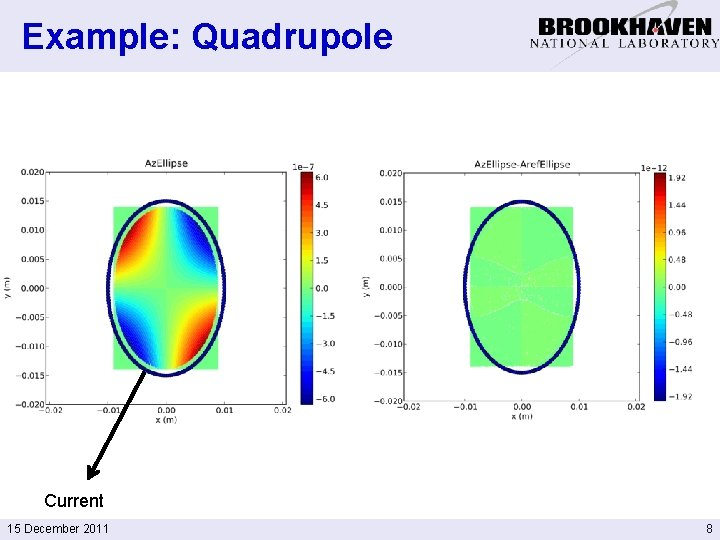Example: Quadrupole Current 15 December 2011 8Rectangular Shape Conductor 15 December 2011 Reference Az 9From 2 D to 3 D • Power each current strand individually Vector addition – Very inefficient, clumsy – Not very elegant • Known current distribution • Helical coil: vector addition of two currents, which always intersect at the correct angle 15 December 2011 10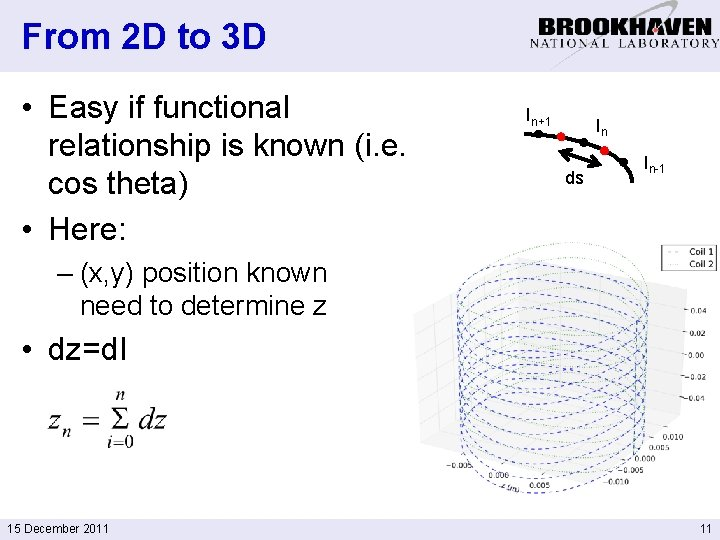From 2 D to 3 D • Easy if functional relationship is known (i. e. cos theta) • Here: In+1 In ds In-1 – (x, y) position known need to determine z • dz=d. I 15 December 2011 11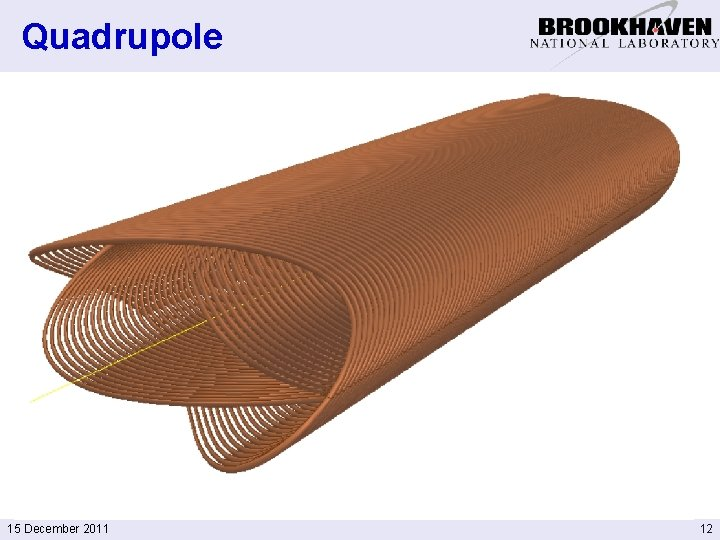Quadrupole 15 December 2011 12Quadrupole Calculated for two coils 15 December 2011 13Task Inside pipe width = 5 cm Inside pipe height = 2 cm From: Suggested shield & cos theta dipole dimensions R. B. Palmer, 5/26/11 15 December 2011 14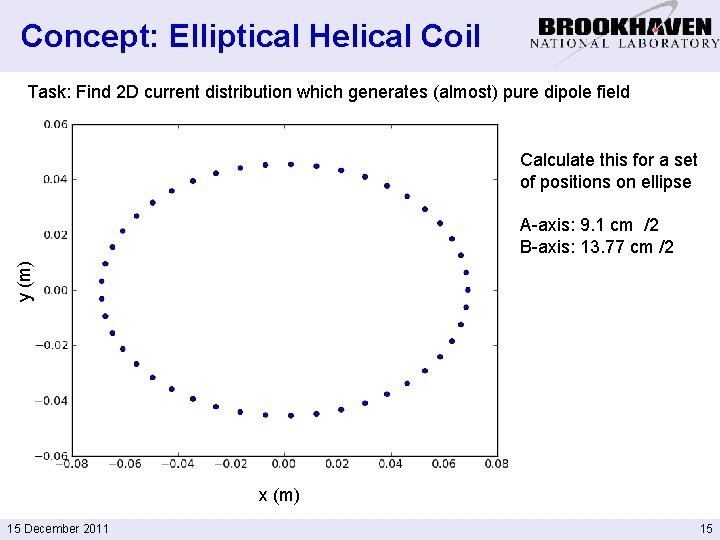Concept: Elliptical Helical Coil Task: Find 2 D current distribution which generates (almost) pure dipole field Calculate this for a set of positions on ellipse y (m) A-axis: 9. 1 cm /2 B-axis: 13. 77 cm /2 x (m) 15 December 2011 15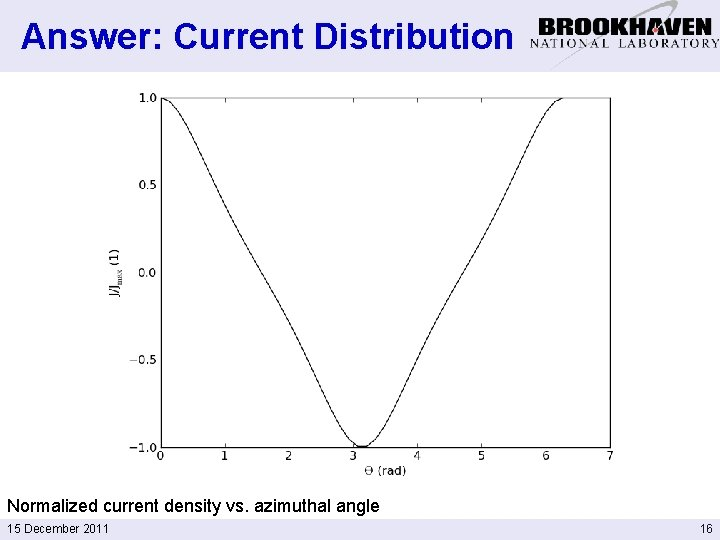Answer: Current Distribution Normalized current density vs. azimuthal angle 15 December 2011 16Implementation: Elliptical Helical Coil 40 turns Spacing: 20 mm (= length about 0. 8 m + “coil ends”) Single double layer Current in strand: 10 k. A (=400 k. A turns) Average current density: 10 k. A/(20 mmx 1 mm)=500 A/mm 2 15 December 2011 17Field Harmonics Normalized to Dipole field of 1 T Evaluated for radius of 25 mm Well behaved: small sextupole component at coil entrance and exit 15 December 2011 18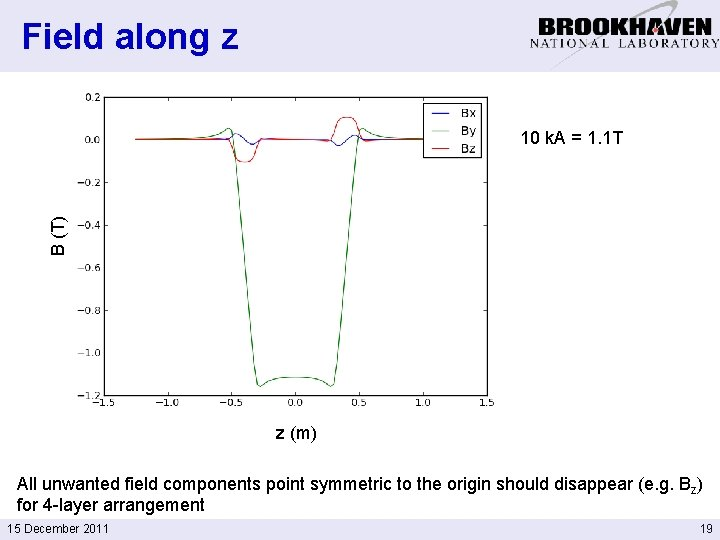Field along z B (T) 10 k. A = 1. 1 T z (m) All unwanted field components point symmetric to the origin should disappear (e. g. Bz) for 4 -layer arrangement 15 December 2011 19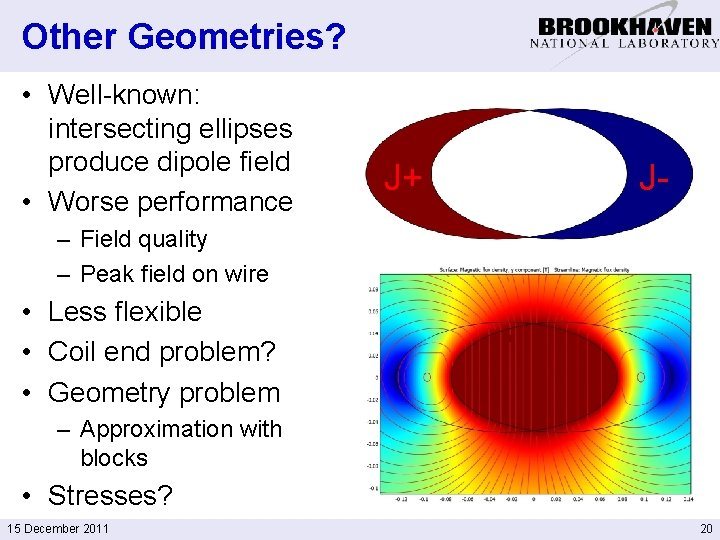Other Geometries? • Well-known: intersecting ellipses produce dipole field • Worse performance J+ J- – Field quality – Peak field on wire • Less flexible • Coil end problem? • Geometry problem – Approximation with blocks • Stresses? 15 December 2011 20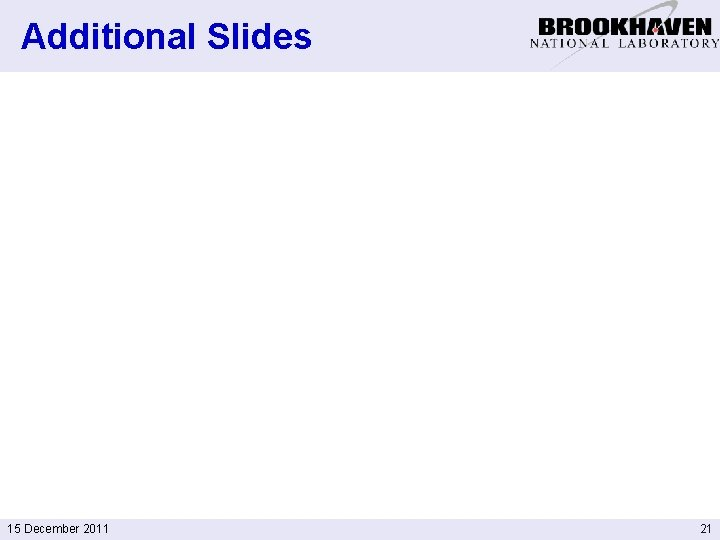Additional Slides 15 December 2011 21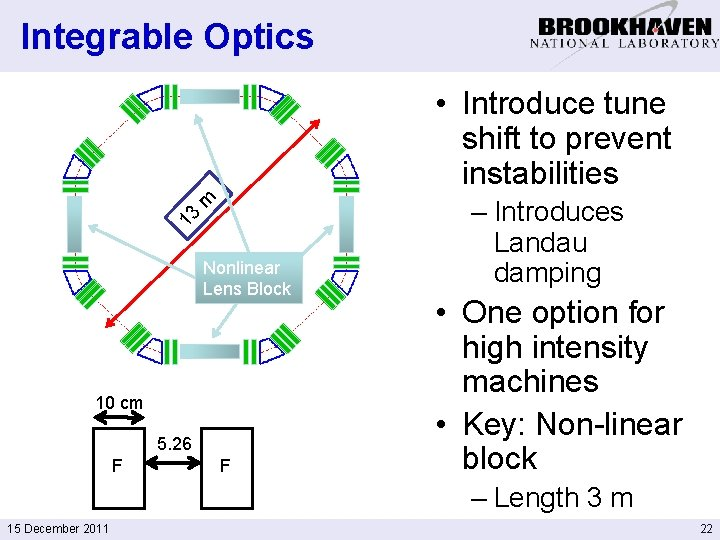Integrable Optics 13 m • Introduce tune shift to prevent instabilities Nonlinear Lens Block 10 cm 5. 26 F F – Introduces Landau damping • One option for high intensity machines • Key: Non-linear block – Length 3 m 15 December 2011 22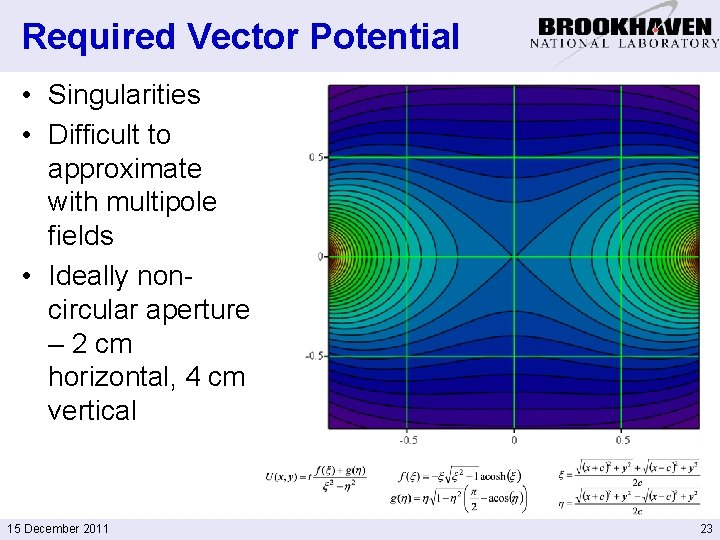Required Vector Potential • Singularities • Difficult to approximate with multipole fields • Ideally noncircular aperture – 2 cm horizontal, 4 cm vertical 15 December 2011 23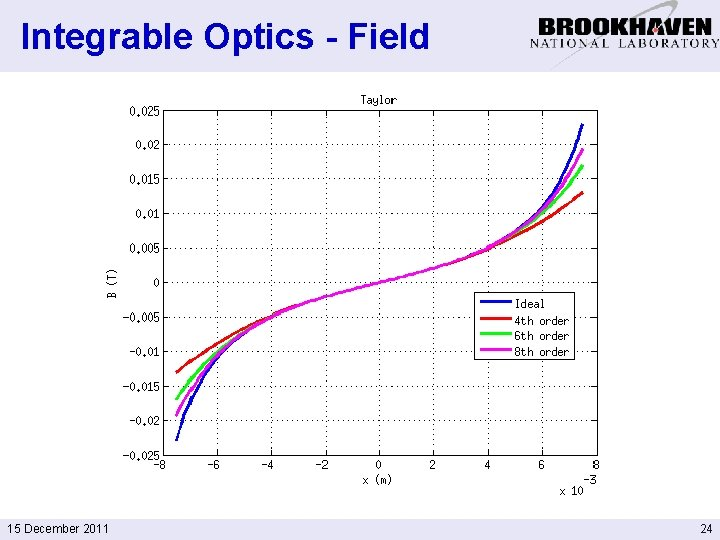Integrable Optics - Field 15 December 2011 24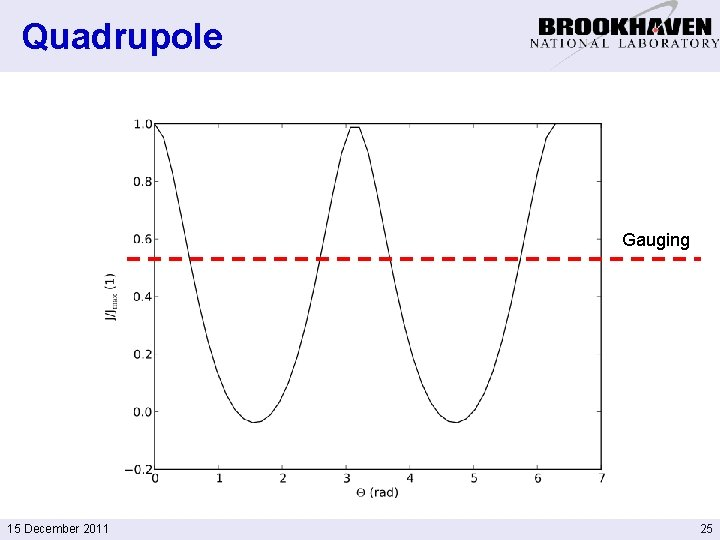Quadrupole Gauging 15 December 2011 25Gauging • Circular coil: constant current in longitudinal direction will cause a uniform vector potential A 0 within this circle • Az(x, y)=A 1(x, y)+A 0 • N. b. : • Ergo: changes vector potential but not field • Allows to shift current 15 December 2011 26Gauging for elliptical coils • For elliptical coils (or other shapes): some modest variation of Az • Example: quadrupole • Correction per current strand: 2 k. A • Field: 0. 3 m. T 15 December 2011 27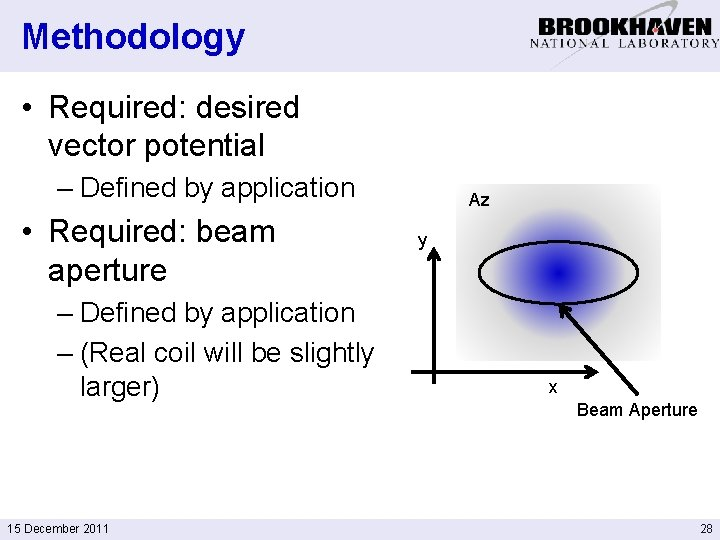Methodology • Required: desired vector potential – Defined by application • Required: beam aperture – Defined by application – (Real coil will be slightly larger) 15 December 2011 Az y x Beam Aperture 28Methodology (cont. ) • Define point P 1 on desired crosssection (known Az) • Define current I 1 (for example on coil cross-section) • Az can be calculated from 15 December 2011 I 1 P 1 29